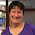### Google Script - Setting up Reminder Dates

A person in my department keeps track of software subscriptions, and asked if there was a way to automatically have it provide a reminder 45 days prior to the renewal dates.  I said sure! Once I set up the addition of a "reminder date", I modified an existing script to have it write that to her calendar.  So now she simply inputs the vendor information, purchase date & renewal date, and then the scripts calculate the reminder date and adds it to the calendar.  It seems to work pretty slick!

Here are the steps to create the reminder dates with a script -

This can be a stand-alone spreadsheet, or it can be one that is populated by a form.  Here is my sample-

2. Open up the Script editor
Go to the Tools Menu and select Script editor

3.  Replace the code
Replace the default code -

with this - (all the text highlighted in gray include the final bracket)
var dateIndex = 6; //cell with reference date

var sheet = ss.getSheets();

var lastRow = sheet.getLastRow();
var lastColumn = sheet.getLastColumn();

function setDate() {

var data = sheet.getRange(1, 1, lastRow, lastColumn).getValues();

for (var i = 1; i < data.length; ++i) {
var row = data[i];
var renewal = sheet.getRange(i+1, dateIndex, 1, 1).getValue();  //renewal date
var reminder = sheet.getRange(i+1, 11, 1, 1);  //cell to put in reminder date

if (renewal !== "-"){

ND2 = new Date(renewal.setDate(renewal.getDate()-45));  //takes value of date and subtracts 45 days for reminder

reminder.setValue(ND2)  //sets reminder date to new date

}}
}

Let's break it down.  First, I'm establishing some variables - the column that has the reference date I will need later, then choosing which spreadsheet & sheet I am using, and last establishing the number value of the last column and row in the spreadsheet.

var dateIndex = 6;  //cell with reference date

var sheet = ss.getSheets();

var lastRow = sheet.getLastRow();
var lastColumn = sheet.getLastColumn();

Next I named my function, and "grabbed" the values from the spreadsheet -
function setDate() {

var data = sheet.getRange(1, 1, lastRow, lastColumn).getValues();

Next I created my loop so that it would look at each row in the spreadsheet, and established the variables that I am only going to use within the loop -

for (var i = 1; i < data.length; ++i) {
var row = data[i];
var renewal = sheet.getRange(i+1, dateIndex, 1, 1).getValue();  //re
newal date
var reminder = sheet.getRange(i+1, 11, 1, 1);  //cell to put in reminder date

I put in an if statement to only calculate the reminder date if there was a renewal date -

if (renewal !== "-"){

After that, I added the line to subtract 45 days from the renewal date:

ND2 = new Date(renewal.setDate(renewal.getDate()-45));  //takes value of date and subtracts 45 days for reminder

Now you can use this in many different ways - I think changing the number of days is the easiest to figure out because you can use the getDate (for the day) and then add or subtract.

Last I assigned that value to the reminder date in that row -

reminder.setValue(ND2)  //sets reminder date to new date

That's it!  Make sure to pay attention to your curly brackets when using this.  And, if you want it automatic, make sure to set up a trigger for it to run (under the script edit menu). Once I run the script, you will notice that the reminder dates are set, and those that do not have renewal dates are blank -
This sets it up so I can then create my next script to make calendar events to remind me of upcoming renewal dates.

As an FYI - I did a similar one where I needed to add minutes - a bit more work than changing the days, but thanks to this entry by Serge insas in StackOverflow you can see how to manipulate any of the time elements by using the getTime - you do have to figure out the correct multipliers, though, for minutes, seconds, hours, etc.

Tomorrow I'll share the script that writes it to the calendar.

1.Hello Melinda, I'm sorry but I'm a bit of a newbie to scripts and it looks like I've made a mistake on my first attempt. I don't have a clue how to rectify it because I don't understand what I'm doing! I copied in your script and received this red message... TypeError: Cannot find function setDate in object. Do you know what I need to do?

2.Ignore my last post! I've just read another of your guides and you mention that, if the data is not on the first sheet I have to add the number of the sheet I want to use. So, instead of var sheet = ss.getSheets(); (which is the first sheet in the spreadsheet) I used var sheet = ss.getSheets(); (to use the next sheet) and voila! It works! Thank you!!!

1.Glad you got it to work!